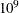# The OPTMILP Procedure

### ODS Tables

PROC OPTMILP creates three Output Delivery System (ODS) tables by default. The first table, ProblemSummary, is a summary of the input MILP problem. The second table, SolutionSummary, is a brief summary of the solution status. The third table, PerformanceInfo, is a summary of performance options. You can use ODS table names to select tables and create output data sets. For more information about ODS, see SAS Output Delivery System: User's Guide.

If you specify a value of 2 for the PRINTLEVEL= option, then the ProblemStatistics table is produced. This table contains information about the problem data. See the section Problem Statistics for more information.

If you specify the DETAILS option in the PERFORMANCE statement, then the Timing table is produced.

Table 11.13 lists all the ODS tables that can be produced by the OPTMILP procedure, along with the statement and option specifications required to produce each table.

Table 11.13: ODS Tables Produced by PROC OPTMILP

ODS Table Name

Description

Statement

Option

ProblemSummary

Summary of the input MILP problem

PROC OPTMILP

PRINTLEVEL=1 (default)

SolutionSummary

Summary of the solution status

PROC OPTMILP

PRINTLEVEL=1 (default)

ProblemStatistics

Description of input problem data

PROC OPTMILP

PRINTLEVEL=2

PerformanceInfo

List of performance options and their values

PROC OPTMILP

PRINTLEVEL=1 (default)

Timing

Detailed solution timing

PERFORMANCE

DETAILS

A typical ProblemSummary table is shown in Figure 11.5.

Figure 11.5: Example PROC OPTMILP Output: Problem Summary

The OPTMILP Procedure

Problem Summary
Problem Name EX_MIP
Objective Sense Minimization
Objective Function COST
RHS RHS

Number of Variables 3
Bounded Above 0
Bounded Below 0
Bounded Above and Below 3
Free 0
Fixed 0
Binary 3
Integer 0

Number of Constraints 3
LE (<=) 2
EQ (=) 0
GE (>=) 1
Range 0

Constraint Coefficients 8

A typical SolutionSummary table is shown in Figure 11.6.

Figure 11.6: Example PROC OPTMILP Output: Solution Summary

The OPTMILP Procedure

Solution Summary
Solver MILP
Algorithm Branch and Cut
Objective Function COST
Solution Status Optimal
Objective Value -7

Relative Gap 0
Absolute Gap 0
Primal Infeasibility 0
Bound Infeasibility 0
Integer Infeasibility 0

Best Bound .
Nodes 0
Iterations 0
Presolve Time 0.00
Solution Time 0.00

You can create output data sets from these tables by using the ODS OUTPUT statement. The output data sets from the preceding example are displayed in Figure 11.7 and Figure 11.8, where you can also find variable names for the tables used in the ODS template of the OPTMILP procedure.

Figure 11.7: ODS Output Data Set: Problem Summary

 Problem Summary

Obs Label1 cValue1 nValue1
1 Problem Name EX_MIP .
2 Objective Sense Minimization .
3 Objective Function COST .
4 RHS RHS .
5     .
6 Number of Variables 3 3.000000
7 Bounded Above 0 0
8 Bounded Below 0 0
9 Bounded Above and Below 3 3.000000
10 Free 0 0
11 Fixed 0 0
12 Binary 3 3.000000
13 Integer 0 0
14     .
15 Number of Constraints 3 3.000000
16 LE (<=) 2 2.000000
17 EQ (=) 0 0
18 GE (>=) 1 1.000000
19 Range 0 0
20     .
21 Constraint Coefficients 8 8.000000

Figure 11.8: ODS Output Data Set: Solution Summary

 Solution Summary

Obs Label1 cValue1 nValue1
1 Solver MILP .
2 Algorithm Branch and Cut .
3 Objective Function COST .
4 Solution Status Optimal .
5 Objective Value -7 -7.000000
6     .
7 Relative Gap 0 0
8 Absolute Gap 0 0
9 Primal Infeasibility 0 0
10 Bound Infeasibility 0 0
11 Integer Infeasibility 0 0
12     .
13 Best Bound . .
14 Nodes 0 0
15 Iterations 0 0
16 Presolve Time 0.02 0.015600
17 Solution Time 0.02 0.015600

#### Problem Statistics

Optimizers can encounter difficulty when solving poorly formulated models. Information about data magnitude provides a simple gauge to determine how well a model is formulated. For example, a model whose constraint matrix contains one very large entry (on the order of) can cause difficulty when the remaining entries are single-digit numbers. The PRINTLEVEL=2 option in the OPTMILP procedure causes the ODS table ProblemStatistics to be generated. This table provides basic data magnitude information that enables you to improve the formulation of your models.

The example output in Figure 11.9 demonstrates the contents of the ODS table ProblemStatistics.

Figure 11.9: ODS Table ProblemStatistics

 ProblemStatistics

Obs Label1 cValue1 nValue1
1 Number of Constraint Matrix Nonzeros 8 8.000000
2 Maximum Constraint Matrix Coefficient 3 3.000000
3 Minimum Constraint Matrix Coefficient 1 1.000000
4 Average Constraint Matrix Coefficient 1.875 1.875000
5     .
6 Number of Objective Nonzeros 3 3.000000
7 Maximum Objective Coefficient 4 4.000000
8 Minimum Objective Coefficient 2 2.000000
9 Average Objective Coefficient 3 3.000000
10     .
11 Number of RHS Nonzeros 3 3.000000
12 Maximum RHS 7 7.000000
13 Minimum RHS 4 4.000000
14 Average RHS 5.3333333333 5.333333
15     .
16 Maximum Number of Nonzeros per Column 3 3.000000
17 Minimum Number of Nonzeros per Column 2 2.000000
18 Average Number of Nonzeros per Column 2 2.000000
19     .
20 Maximum Number of Nonzeros per Row 3 3.000000
21 Minimum Number of Nonzeros per Row 2 2.000000
22 Average Number of Nonzeros per Row 2 2.000000

The variable names in the ODS table ProblemStatistics are Label1, cValue1, and nValue1.